# Data Transformation (Part 1) | Normalisation Techniques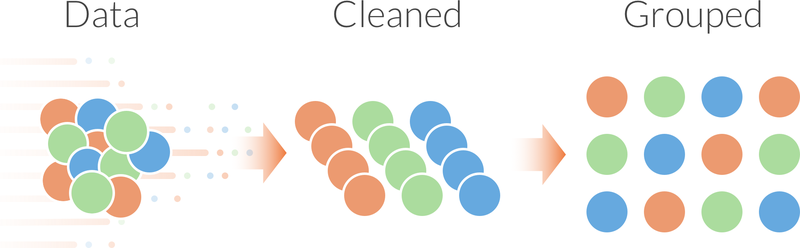Almost always when we get raw data in any project, it is unfit for direct consumption for analysis or modelling . It is a especially a concern when the data volume is huge for example in a big data analytics project . In this blog post I cover a few of the most common transformations and their use.

#### Normalisation

The process of normalization entails converting numerical values into a new range using a mathematical function. There are two primary reasons why this may be used.

##### To make two variables in different scales comparable

In a profile of a customer where I may have two variables - years of education and income. We might want both these to be treated equally but their ranges are very different. Plotting them on a graph may make it impossible to decipher any correlation between these two variables. However, normalization would bring them on to the scale and the relationship would clearly stand out.

##### Some models may need the data to be normalized before modeling

KNN models, for example, require a pre-requisite normalization for the model to produce effective results. Refer to this article for greater details.

Some common normalization methods are as follows.

##### Min-Max

Min-Max is probably the most commonly used transformation. This transforms the numerical variable into a new range, for example, 0 to 1. It is calculated by the formula given below.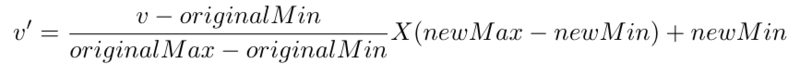For example, consider the range of marks that a set of students have scored by roll number given below

Roll NumberMarks
110
215
350
460

If we were to normalize it between the ranges of 0 to 1 we would get the following

Roll NumberCalculationNormalised marks
1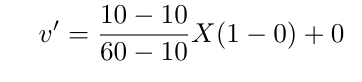0
2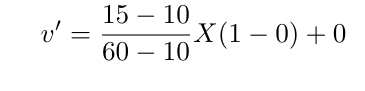.1
3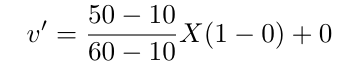.8
4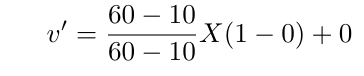1

As we can see above that we have taken max as the maximum marks as obtained by the student as opposed to the maximum marks possible. However if from the original data set, it is possible to determine the maximum ranges then that is what we should be using and 60 in the above formulae should have been replaced by 100.

z-score
Simply put the z-score is the number of standard deviations a data point is from the mean of the data set. To be able to understand this we must understand what is standard deviation. The formula for z-score is as below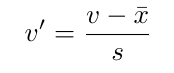where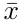is the mean andis the standard deviation

For the above data set the z-score calculation of each observation is as follows.

mean is 33.75 and Standard Deviation is 24.95

Roll NumberMarksZ-Score
110-0.7037037037
215-0.5555555556
3500.4814814815
4600.7777777778

##### Box-Cox Transformation

It is not necessary for a data set to adhere to a normal distribution. However many data analysis methods require the data distribution to be normal. Box-Cox is a transformation that can be used to convert any distribution to a normal distribution. Every dataset may not benefit from a Box-Cox transformation, for example, if there are significant outliers box-cos may not help.

The box-cox transformation in mathematical form is denoted as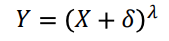where λ is the exponent (power) and δ is a shift amount that is added when X is zero or negative. When λ is zero, the above definition is replaced byAs you can very well imagine the trick is to find the right value of λ to get a normal distribution.

Usually, the standard λ values of -2, -1.5, -1, -0.5, 0, 0.5, 1, 1.5, and 2 are investigated to determine which, if any, is most suitable. However, a maximum likelihood estimation can be used to determine the best possible value of λ to get a more normal distribution.

To understand Box-Cox transformation lets look at a non normal data-set and see the impact of the transformation on it.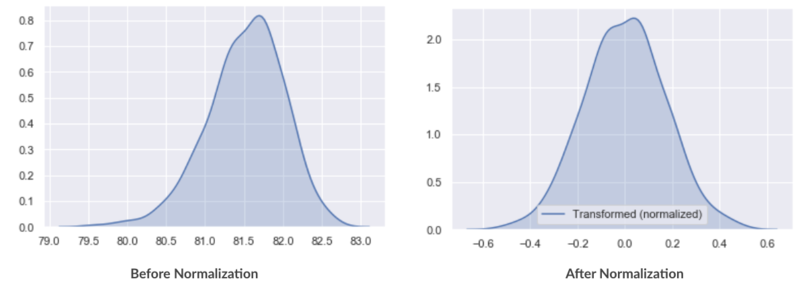In part II of this series of normalisation, we will discuss Aggregations, Value Mapping and Discretization.